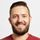cancel
Showing results for
Did you mean:Frequent Visitor

## Month on Month at Day granularity repeating values

I'm doing month on month comparison on a day level. so column 1 is May and column 2 is April's figures however you can see on May 31st the value for April is being duplicated. I would have expected BLANK as there is no April 31st. Please see below

```GP PM Test =
CALCULATE (
[GP],
)```If I use previous month or parallel period when unfiltered or filtered for specific dates it returns the whole months total for everyday as seen here. When I select a full Month it still repeats for May 31st where I would have expected a blank

```GP PM Test =
CALCULATE (
[GP],
PREVIOUSMONTH('Calendar'[Date])
)```2 ACCEPTED SOLUTIONSMicrosoft

```GP PM Test =
CALCULATE (
[GP],
PARALLELPERIOD ( 'Calendar'[Datekey], -1, MONTH ),
FILTER (
ALL ( 'Calendar' ),
DAY ( 'Calendar'[Datekey] ) = DAY ( MAX ( 'Calendar'[Datekey] ) )
)
)
```Best Regards,

Dale

Community Support Team _ Dale
If this post helps, then please consider Accept it as the solution to help the other members find it more quickly.Microsoft

According to my understandings, the part is evaluated by the row context and the filter context. The filter context is from the visual. The blue part will iterate row by row of the 'Calendar', which means the row context.

```FILTER (
ALL ( 'Calendar' ),
DAY ( 'Calendar'[Datekey] ) = DAY ( MAX ( 'Calendar'[Datekey] ) )
)```

For example, the day 5/30/2018. The MAX function always ignore the row context which is from "All('Calendar')". So the right side is 30. Then the blue part will be iterated row by row of the Calendar table. When the whole blue part is true, the rows will be chosen.

Reference:

https://www.microsoftpressstore.com/articles/article.aspx?p=2449191 (There are always two contexts)

https://msdn.microsoft.com/en-us/query-bi/dax/filter-function-dax

Best Regards,

Dale

Community Support Team _ Dale
If this post helps, then please consider Accept it as the solution to help the other members find it more quickly.
3 REPLIES 3Microsoft

```GP PM Test =
CALCULATE (
[GP],
PARALLELPERIOD ( 'Calendar'[Datekey], -1, MONTH ),
FILTER (
ALL ( 'Calendar' ),
DAY ( 'Calendar'[Datekey] ) = DAY ( MAX ( 'Calendar'[Datekey] ) )
)
)
```Best Regards,

Dale

Community Support Team _ Dale
If this post helps, then please consider Accept it as the solution to help the other members find it more quickly.Frequent Visitor

Thanks @v-jiascu-msft, I should have mentioned I already have a measure that works, with similar logic. Yours is slightly simpler, but it breaks my head 🙂 do you mind explaining the filter part. I can see it looks at the whole calendar and then filters the calenedar where the days are equal but this is where I'm getting lost.

`DAY ( 'Calendar'[Datekey] ) = DAY ( MAX ( 'Calendar'[Datekey] ) )`

Does the left side refere to each date in the calendar and then the right side is the MAX of the whole calendar?Microsoft

According to my understandings, the part is evaluated by the row context and the filter context. The filter context is from the visual. The blue part will iterate row by row of the 'Calendar', which means the row context.

```FILTER (
ALL ( 'Calendar' ),
DAY ( 'Calendar'[Datekey] ) = DAY ( MAX ( 'Calendar'[Datekey] ) )
)```

For example, the day 5/30/2018. The MAX function always ignore the row context which is from "All('Calendar')". So the right side is 30. Then the blue part will be iterated row by row of the Calendar table. When the whole blue part is true, the rows will be chosen.

Reference:

https://www.microsoftpressstore.com/articles/article.aspx?p=2449191 (There are always two contexts)

https://msdn.microsoft.com/en-us/query-bi/dax/filter-function-dax

Best Regards,

Dale

Community Support Team _ Dale
If this post helps, then please consider Accept it as the solution to help the other members find it more quickly.